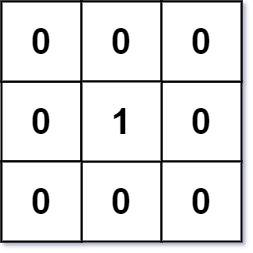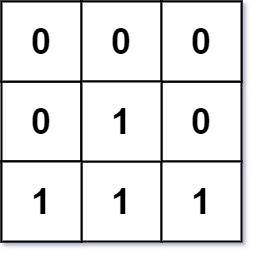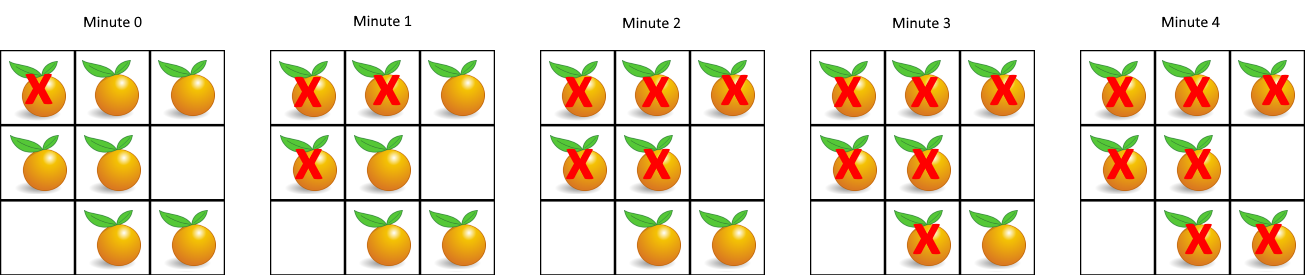# 2022-05-23

## ​542. 01 Matrix​

### Description

Given an `m x n` binary matrix `mat`, return the distance of the nearest `0` for each cell.
The distance between two adjacent cells is `1`.
Example 1:Input: mat = [[0,0,0],[0,1,0],[0,0,0]]
Output: [[0,0,0],[0,1,0],[0,0,0]]
Example 2:Input: mat = [[0,0,0],[0,1,0],[1,1,1]]
Output: [[0,0,0],[0,1,0],[1,2,1]]
Constraints:
• `m == mat.length`
• `n == mat[i].length`
• `1 <= m, n <= 10^4`
• `1 <= m * n <= 10^4`
• `mat[i][j]` is either `0` or `1`.
• There is at least one `0` in `mat`.

### Solution

#### Approach #0: BFS (Time Limit Exceeded) ❌

var (
dx = []int{0, 1, 0, -1}
dy = []int{1, 0, -1, 0}
)
func updateMatrix(mat [][]int) [][]int {
if len(mat) == 0 {
return mat
}
m, n := len(mat), len(mat)
res := make([][]int, m)
for i := 0; i < m; i++ {
res[i] = make([]int, n)
}
for i := 0; i < m; i++ {
for j := 0; j < n; j++ {
queue := [][]int{{i, j}}
depth := -1
t := make([][]int, m)
for i := 0; i < m; i++ {
t[i] = make([]int, n)
}
for len(queue) > 0 {
size := len(queue)
for k := 0; k < size; k++ {
x, y := queue[k], queue[k]
t[x][y] = 1
if mat[x][y] == 0 {
queue = queue[:0]
break
} else {
for p := 0; p < 4; p++ {
xx, yy := x+dx[p], y+dy[p]
if xx >= 0 && xx < m && yy >= 0 && yy < n && t[xx][yy] != 1 {
queue = append(queue, []int{xx, yy})
}
}
}
}
depth++
if len(queue) > 0 {
queue = queue[size:]
}
}
res[i][j] = depth
}
}
return res
}

#### Approach #1: BFS

var (
dx = []int{0, 1, 0, -1}
dy = []int{1, 0, -1, 0}
)
func updateMatrix(mat [][]int) [][]int {
if len(mat) == 0 {
return mat
}
m, n := len(mat), len(mat)
res := make([][]int, m)
checked := make([][]int, m)
for i := 0; i < m; i++ {
res[i] = make([]int, n)
checked[i] = make([]int, n)
}
var queue [][]int
for i := 0; i < m; i++ {
for j := 0; j < n; j++ {
if mat[i][j] == 0 {
queue = append(queue, []int{i, j})
checked[i][j] = 1
}
}
}
depth := 0
for len(queue) > 0 {
size := len(queue)
for i := 0; i < size; i++ {
x, y := queue[i], queue[i]
res[x][y] = depth
for p := 0; p < 4; p++ {
xx, yy := x+dx[p], y+dy[p]
if xx >= 0 && xx < m && yy >= 0 && yy < n && checked[xx][yy] != 1 {
checked[xx][yy] = 1
queue = append(queue, []int{xx, yy})
}
}
}
depth++
queue = queue[size:]
}
return res
}

## ​994. Rotting Oranges​

### Description

You are given an `m x n` `grid` where each cell can have one of three values:
• `0` representing an empty cell,
• `1` representing a fresh orange, or
• `2` representing a rotten orange.
Every minute, any fresh orange that is 4-directionally adjacent to a rotten orange becomes rotten.
Return the minimum number of minutes that must elapse until no cell has a fresh orange. If this is impossible, return `-1`.
Example 1:Input: grid = [[2,1,1],[1,1,0],[0,1,1]]
Output: 4
Example 2:
Input: grid = [[2,1,1],[0,1,1],[1,0,1]]
Output: -1
Explanation: The orange in the bottom left corner (row 2, column 0) is never rotten, because rotting only happens 4-directionally.
Example 3:
Input: grid = [[0,2]]
Output: 0
Explanation: Since there are already no fresh oranges at minute 0, the answer is just 0.
Constraints:
• `m == grid.length`
• `n == grid[i].length`
• `1 <= m, n <= 10`
• `grid[i][j]` is `0`, `1`, or `2`.

### Solution

#### Approach #0: BFS

var (
dx = []int{0, 1, 0, -1}
dy = []int{1, 0, -1, 0}
)
func orangesRotting(grid [][]int) int {
if len(grid) == 0 {
return 0
}
m, n := len(grid), len(grid)
all1 := 0
var queue [][]int
for i := 0; i < m; i++ {
for j := 0; j < n; j++ {
if grid[i][j] == 1 {
all1++
}
if grid[i][j] == 2 {
queue = append(queue, []int{i, j})
}
}
}
if len(queue) == 0 && all1 == 0 {
return 0
}
depth := -1
count1 := -len(queue)
for len(queue) > 0 {
size := len(queue)
for i := 0; i < size; i++ {
x, y := queue[i], queue[i]
count1++
for j := 0; j < 4; j++ {
xx, yy := x+dx[j], y+dy[j]
if xx >= 0 && xx < m && yy >= 0 && yy < n && grid[xx][yy] == 1 {
grid[xx][yy] = 2
queue = append(queue, []int{xx, yy})
}
}
}
fmt.Println(all1, count1)
depth++
queue = queue[size:]
}
if all1 > count1 {
return -1
}
return depth
}